MANGANESE

```
```

This page looks at some aspects of manganese chemistry required for UK A' level exams. It includes: two simple reactions of manganese(II) ions in solution (summarised from elsewhere on the site), and the use of potassium manganate(VII) (potassium permanganate) as an oxidising agent - including its use in titrations.

```
```

Reactions of manganese(II) ions in solution

The simplest ion that manganese forms in solution is the hexaaquamanganese(II) ion - [Mn(H2O)6]2+.

Note:  If you aren't happy about complex ions (including the way they are bonded and named), it would pay you to follow this link and explore the first couple of pages in the complex ions menu before you go on.

The reaction of hexaaquamanganese(II) ions with hydroxide ions

Hydroxide ions (from, say, sodium hydroxide solution) remove hydrogen ions from the water ligands attached to the manganese ion.

Once a hydrogen ion has been removed from two of the water molecules, you are left with a complex with no charge - a neutral complex. This is insoluble in water and a precipitate is formed.Note:  The colour coding is to show that this isn't a ligand exchange reaction. The oxygens which were originally attached to the manganese are still attached in the neutral complex.

In the test-tube, the colour changes are:I have shown the original solution as very pale pink (the palest I can produce!), but in fact it is virtually colourless. The pale brown precipitate is oxidised to a darker brown manganese(III) compound in contact with oxygen from the air.

Note:  You will find the reactions between hexaaqua ions and hydroxide ions discussed in detail if you follow this link.

There is a lot of disagreement in the literature about what the darker brown compound is. It is most commonly quoted (including in a couple of research papers) as MnO(OH) or sometimes as Mn2O3,xH2O.

However, some sources quote it as a hydrated manganese(IV) oxide, MnO2,xH2O. One of the UK Exam Boards (Edexcel International) had this as a part of a question in January 2015.

The reaction of hexaaquamanganese(II) ions with ammonia solution

Ammonia can act as both a base and a ligand. In this case, at usual lab concentrations, it simply acts as a base - removing hydrogen ions from the aqua complex.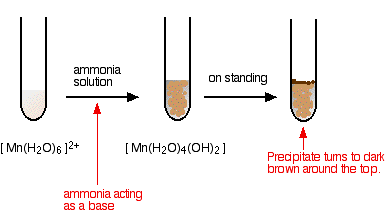Again, I have shown the original solution as the palest pink I can produce, but in fact it is virtually colourless. The pale brown precipitate is oxidised to a darker brown manganese(III) compound in contact with oxygen from the air.

There is no observable difference in appearance between this reaction and the last one.

Note:  You will find the reactions between hexaaqua ions and ammonia solution discussed in detail if you follow this link.

```
```

Some potassium manganate(VII) chemistry

Potassium manganate(VII) (potassium permanganate) is a powerful oxidising agent.

Using potassium manganate(VII) as an oxidising agent in organic chemistry

Potassium manganate(VII) is usually used in neutral or alkaline solution in organic chemistry. Acidified potassium manganate(VII) tends to be a rather destructively strong oxidising agent, breaking carbon-carbon bonds.

The potassium manganate(VII) solution is usually made mildly alkaline with sodium carbonate solution, and the typical colour changes are:In testing for a C=C double bond

Potassium manganate(VII) oxidises carbon-carbon double bonds, and so goes through the colour changes above.

Ethene, for example, is oxidised to ethane-1,2-diol.The oxygen in square brackets is taken to mean "oxygen from an oxidising agent". This abbreviated form of the equation is most commonly used in organic chemistry. You are very unlikely to have to write the complete ionic equation for this reaction at this level.

To be honest, this isn't a good test for a carbon-carbon double bond, because anything which is even mildly reducing would have the same effect on the potassium manganate(VII) solution.

You could, however, use this reaction simply as a means of making the diol.

In the oxidation of aromatic side chains

Alkaline potassium manganate(VII) solution oxidises any hydrocarbon side chain attached to a benzene ring back to a single -COOH group. Prolonged heating is necessary.

For example: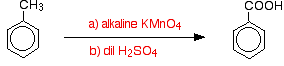Because the solution is alkaline, you will initially get benzoate ions formed rather than the acid. The sulphuric acid is added to convert the ions to the acid.

With longer side chains, you will get all sorts of mixtures of other products - but in each case, the main product will be benzoic acid.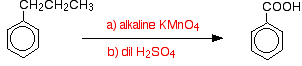```
```

Using potassium manganate(VII) as an oxidising agent in titrations

Background

Potassium manganate(VII) solution is used to find the concentration of all sorts of reducing agents. It is always used in acidic solution.

For example, it oxidises

• iron(II) ions to iron(III) ions• hydrogen peroxide solution to oxygen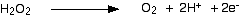• ethanedioic acid to carbon dioxide (This reaction has to be done hot.)• sulphite ions (sulphate(IV) ions) to sulphate ions (sulphate(VI) ions)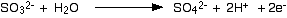In each case, the half-equation for the manganate(VII) ions in acidic solution is:These equations can be combined to give you an overall ionic equation for each possible reaction. That, of course, also gives you the reacting proportions.

For example, when the equations are combined, you find that 1 mole of MnO4- ions react with 5 moles of Fe2+ ions. Having got that information, the titration calculations are just like any other ones.

Note:  If you don't know how to work out half-equations and combine them into equations like this, you can find out how to do it on the page about writing ionic equations for redox reactions.

If you aren't very good at titration calculations, you might be interested in my chemistry calculations book.

Doing the titration

The potassium manganate(VII) solution always goes into the burette, and the other solution in the flask is acidified with dilute sulphuric acid.

As the potassium manganate(VII) solution is run into the flask it becomes colourless. The end point is the first faint trace of permanent pink in the solution showing that there is a tiny excess of manganate(VII) ions present.

Problems with the use of potassium manganate(VII) solution

There are two things you need to be aware of:

• Potassium manganate(VII) can't be used in titrations in the presence of ions like chloride or bromide which it oxidises. An unknown amount of the potassium manganate(VII) would be used in side reactions, and so the titration result would be inaccurate.

This is why you don't acidify the solution with hydrochloric acid.

• Potassium manganate(VII) isn't a primary standard. That means that it can't be made up to give a stable solution of accurately known concentration.

It is so strongly coloured that it is impossible to see when all the crystals you have used have dissolved, and over a period of time it oxidises the water it is dissolved in to oxygen.

Bottles of potassium manganate(VII) solution usually have a brown precipitate around the top. This is manganese(IV) oxide - and is produced when the manganate(VII) ions react with the water.

You have to make up a solution which is approximately what you want, and then standardise it by doing a titration. This is often done with ethanedioic acid solution, because this is a primary standard.

```
```
 Questions to test your understanding If this is the first set of questions you have done, please read the introductory page before you start. You will need to use the BACK BUTTON on your browser to come back here afterwards. questions on manganese answers
```
```

Where would you like to go now?

To the transition metal menu . . .

To the Inorganic Chemistry menu . . .

To Main Menu . . .

```
```